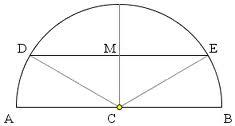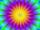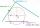# Chord

In a circle with radius r=60 cm is chord 4× longer than its distance from the center. What is the length of the chord?

t =  107.3 cm

### Step-by-step explanation:Did you find an error or inaccuracy? Feel free to write us. Thank you!Tips to related online calculators
Do you want to convert length units?
Pythagorean theorem is the base for the right triangle calculator.

#### You need to know the following knowledge to solve this word math problem:

We encourage you to watch this tutorial video on this math problem:

## Related math problems and questions:

• Chord ABWhat is the chord AB's length if its distance from the center S of the circle k(S, 92 cm) is 10 cm?
• Circle chordCalculate the length of the chord of the circle with radius r = 10 cm, length of which is equal to the distance from the center of the circle.
• Concentric circles and chordIn a circle with a diameter d = 10 cm, a chord with a length of 6 cm is constructed. What radius have the concentric circle while touch this chord?
• Circle's chordsIn the circle there are two chord length 30 and 34 cm. The shorter one is from the center twice than longer chord. Determine the radius of the circle.
• Circle chordWhat is the length x of the chord circle of diameter 115 m if the distance from the center circle is 11 m?
• Two chordsCalculate the length of chord AB and perpendicular chord BC to circle if AB is 4 cm from the center of the circle and BC 8 cm from the center of the circle.
• Chord distanceThe circle k (S, 6 cm), calculate the chord distance from the center circle S when the length of the chord is t = 10 cm.
• Chord 4I need to calculate the circumference of a circle, I know the chord length c=22 cm and the distance from the center d=29 cm chord to the circle.
• Quarter circleWhat is the radius of a circle inscribed in the quarter circle with a radius of 100 cm?
• The chordCalculate a chord length which the distance from the center of the circle (S, 6 cm) equals 3 cm.
• ChordIt is given to a circle k(r=6 cm) and the points A, B such that / AB / = 8 cm lies on k. Calculate the distance of the center of circle S to the midpoint C of the segment AB.
• TangentsTo circle with a radius of 41 cm from the point R guided two tangents. The distance of both points of contact is 16 cm. Calculate the distance from point R and circle centre.
• Concentric circlesIn the circle with diameter, 19 cm is constructed chord 9 cm long. Calculate the radius of a concentric circle that touches this chord.
• TangentWhat distance is the tangent t of the circle (S, 4 cm) and the chord of this circle, which is 6 cm long and parallel to the tangent t?
• Chord 5It is given circle k / S; 5 cm /. Its chord MN is 3 cm away from the center of the circle . Calculate its length.
• ISO trapezoid v2bases of Isosceles trapezoid measured 20 cm and 4 cm and its perimeter is 55 cm. What is the are of a trapezoid?
• Two parallel chordsIn a circle 70 cm in diameter, two parallel chords are drawn so that the center of the circle lies between the chords. Calculate the distance of these chords if one of them is 42 cm long and the second 56 cm.